## Description of the Hydrogen Atom

A hydrogen atom is electrically neutral, containing a single proton and a single electron bound to the nucleus by the Coulomb force.

### Learning Objectives

Identify the unique features of the hydrogen atom that make it important for calculations in quantum mechanics

### Key Takeaways

#### Key Points

• Atomic hydrogen comprises about 75 percent of the elemental mass of the universe.
• Hydrogen atoms are so reactive that they combine with almost all elements.
• Isolated hydrogen atoms called atomic hydrogen or monatomic hydrogen) are extremely rare; hydrogen is more commonly found in combination with other elements.
• The H–H bond is one of the strongest bonds in chemistry.

#### Key Terms

• quantum field theory: Provides a theoretical framework for constructing quantum mechanical models of systems classically represented by an infinite number of degrees of freedom, that is, fields and many-body systems.
• atom: The smallest possible amount of matter that still retains its identity as a chemical element. Atoms consist of a nucleus surrounded by electrons.
• anisotropic: The property of being directionally dependent, as opposed to isotropy which implies identical properties in all directions.

The hydrogen atom (consisting of one proton and one electron, not the diatomic form H2) has special significance in quantum mechanics and quantum field theory as a simple two-body problem physical system that has yielded many simple analytical solutions in closed-form.

### Modeling the Hydrogen Atom

In 1914, Niels Bohr obtained the spectral frequencies of the hydrogen atom after making a number of simplifying assumptions. These assumptions, the cornerstones of the Bohr model, were not fully correct but did yield the correct energy answers. Bohr’s results for the frequencies and underlying energy values were confirmed by the full quantum-mechanical analysis which uses the Schrödinger equation, as was shown in 1925–1926. The solution to the Schrödinger equation for hydrogen is analytical. From this, the hydrogen energy levels and thus the frequencies of the hydrogen spectral lines can be calculated. The solution of the Schrödinger equation goes much further than the Bohr model, because it also yields the shape of the electron’s wave function ( orbital ) for the various possible quantum-mechanical states, thus explaining the anisotropic character of atomic bonds.A model of the hydrogen atom: This model shows approximate dimensions for nuclear and electron shells (not drawn to scale). It shows a diameter about twice the radius indicated by the Bohr model.

The Schrödinger equation also applies to more complicated atoms and molecules, albeit they rapidly become impossibly difficult beyond hydrogen or other two-body type problems, such as helium cation He+. In most such cases, the solution is not analytical and either computer calculations are necessary or simplifying assumptions must be made.

### Solution of Schrödinger Equation: Overview of Results

The solution of the Schrödinger equation (wave equations) for the hydrogen atom uses the fact that the Coulomb potential produced by the nucleus is isotropic (it is radially symmetric in space and only depends on the distance to the nucleus). Although the resulting energy eigenfunctions (the orbitals) are not necessarily isotropic themselves, their dependence on the angular coordinates follows completely, generally from this isotropy of the underlying potential. The eigenstates of the Hamiltonian (that is, the energy eigenstates) can be chosen as simultaneous eigenstates of the angular momentum operator. This corresponds to the fact that angular momentum is conserved in the orbital motion of the electron around the nucleus. Therefore, the energy eigenstates may be classified by two angular momentum quantum numbers, ℓ and m (both are integers). The angular momentum quantum number ℓ = 0, 1, 2,… determines the magnitude of the angular momentum. The magnetic quantum number m = −,…, +ℓ determines the projection of the angular momentum on the (arbitrarily chosen) z-axis.

In addition to mathematical expressions for total angular momentum and angular momentum projection of wavefunctions, an expression for the radial dependence of the wave functions must be found. It is only here that the details of the 1/r Coulomb potential enter (leading to Laguerre polynomials in r). This leads to a third quantum number, the principal quantum number n = 1, 2, 3,…. The principal quantum number in hydrogen is related to the atom’s total energy. Note the maximum value of the angular momentum quantum number is limited by the principal quantum number: it can run only up to n − 1, i.e. ℓ = 0, 1,…, n − 1.

Due to angular momentum conservation, states of the same ℓ but different m have the same energy (this holds for all problems with rotational symmetry). In addition, for the hydrogen atom, states of the same n but different ℓ are also degenerate (i.e. they have the same energy). However, this is a specific property of hydrogen and is no longer true for more complicated atoms that have a (effective) potential differing from the form 1/r, due to the presence of the inner electrons shielding the nucleus potential.

### Calculated Energy Levels

The energy levels of hydrogen are given by solving the Schrödinger equation for the one-electron atom:

$E = E_{n}=-\frac{e^4m_e}{8\varepsilon _{0}^{2}n^2h^2}$

Empirically, it is useful to group the fundamental constants into Rydbergs, which gives the much simpler equation below that turns out to be identical to that predicted by Bohr theory:

$E_{n}=-\frac{1}{n^2}{Ry}$

Further derivation can be performed to include fine structure, which is given by:

$E_{ jn }=\frac { -13.6eV }{ n^{ 2 } } \left[1+\frac { \alpha ^{ 2 } }{ n^{ 2 } } \left( \frac { n }{ j+\frac { 1 }{ 2 } } -\frac { 3 }{ 4 } \right)\right]$

where α is the fine-structure constant and j is a number which is the total angular momentum eigenvalue; that is, ℓ ± 1/2 depending on the direction of the electron spin. The quantity in square brackets arises from relativistic (spin-orbit) coupling interactions.

The value of 13.6 eV is called the Rydberg constant and can be found from the Bohr model and is given by:

$-13.6 eV=-\frac {m_e q_e ^4} {8h^2\epsilon _0^2}$

where me is the mass of the electron, qe is the charge of the electron, h is the Planck constant, and ε0 is the vacuum permittivity.

The Rydberg constant is connected to the fine-structure constant by the relation:

$-13.6 eV=-\frac {m_ec^2\alpha ^2} 2 = - \frac {0.51MeV}{2\cdot 137^2}$

This constant is often used in atomic physics in the form of the Rydberg unit of energy:

$1Ry\equiv hcR_\infty =13.60569253 eV$

## Indeterminacy and Probability Distribution Maps

Quantum indeterminacy refers to the necessary incompleteness in the description of a physical system.

### Learning Objectives

Identify the key distinction between quantum and classical particles with respect to determinism.

### Key Takeaways

#### Key Points

• Quantum indeterminacy is the assertion that the state of a system does not determine a unique collection of values for all its measurable properties.
• The values of an observable will be obtained non-deterministically in accordance with a probability distribution uniquely determined by the system state.
• The units involved in quantum uncertainty are on the order of Planck’s constant (found experimentally to be 6.6 x 10−34 J·s).

#### Key Terms

• observable: Any physical property that can be observed and measured directly and not derived from other properties.
• indeterminacy: The condition of being indeterminate.
• uncertainty: A parameter that measures the dispersion of a range of measured values.

Quantum indeterminacy refers to the necessary incompleteness in the description of a physical system. Indeterminacy has become one of the characteristics of the standard description of quantum physics. Quantum indeterminacy can be quantitatively characterized by a probability distribution on the set of outcomes for measurements of an observable. This distribution is uniquely determined by the system state. Quantum mechanics provides a recipe for calculating this probability distribution.

An adequate account of quantum indeterminacy requires a theory of measurement. Many theories have been proposed since the beginning of quantum mechanics, and quantum measurement continues to be an active research area in both theoretical and experimental physics. Possibly the first systematic attempt at a mathematical theory for quantum measurement was developed by John von Neumann. The measurements he investigated are now called projective measurements.

Quantum indeterminacy can be illustrated in terms of a particle with a definitely measured momentum for which there must be a fundamental limit to how precisely its location can be specified. This quantum uncertainty principle can also be expressed in terms of other variables. For example, a particle with a definitely measured energy has a fundamental limit to how precisely one can specify how long it will have that energy.

$(\Delta{E})(\Delta{t})\geq h$

Therefore the units involved in quantum uncertainty are on the order of Planck’s constant (found experimentally to be 6.6 x 10−34 J·s).

Quantum indeterminacy is the assertion that the state of a system does not determine a unique collection of values for all its measurable properties. In quantum mechanical formalism, it is impossible that, for a given quantum state, each one of these measurable properties (observables) has a determinate (sharp) value. The values of an observable will be obtained non-deterministically in accordance with a probability distribution uniquely determined by the system state. The state is destroyed by measurement, so each measured value in a collection must be obtained using a freshly prepared state.

### Classical Particles

According to classical physics, particles move in a very specific trajectory that is completely determined by the particle’s velocity, position, and the sum of any forces acting on it. In the classical sense, these are known and repeatable. A bullet propelled from a gun at a consistent velocity under identical conditions will always follow the same trajectory and hit the same target. The bullet follows Newton ‘s laws of motion, in which the present determines the future. Newton’s laws are deterministic.

### Quantum Particles

In the world of quantum phenomena, this is not the case. Recall from the uncertainty principle that we cannot simultaneously know an electron ‘s position and velocity—therefore we are unable to determine its trajectory. Since either its present position or velocity is unknown, we cannot know where it will be with any certainty after a known time interval. This is called indeterminacy. We do know where it could be. Based on numerous observations, the quantum state, and the wave equation for the electron, we can determine a statistical map of probable positions for the electron. This is called a probability distribution map, a statistical representation of the probable locations of electrons as they exist in an atom.

The clouds of probability are the locations of electrons as determined by making repeated measurements—each measurement finds the electron in a definite location, with a greater chance of finding the electron in some places rather than others. With repeated measurements, a pattern of probability emerges. The clouds of probability do not look like nor do they correspond to classical orbits. The uncertainty principle prevents us from knowing how the electron gets from one place to another, and so an orbit really does not exist as such. Nature on a small scale is much different from that on the large scale.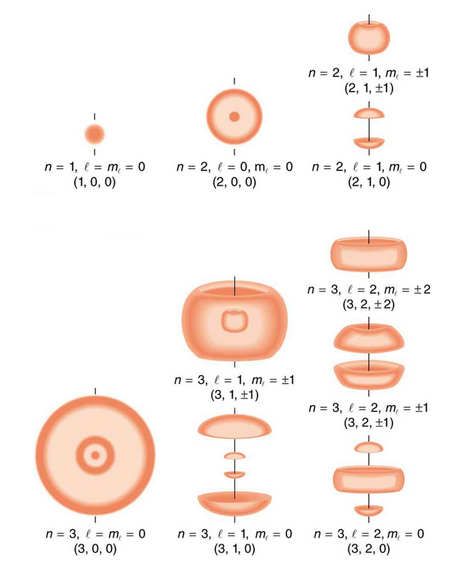Probability density of hydrogen electrons: As indicated by the quantum numbers (n, l, ml), this figure depicts probability clouds for the electron in the ground state and several excited states of hydrogen.

## Quantum Numbers

Quantum numbers provide a numerical description of the orbitals in which electrons reside.

### Learning Objectives

Assign quantum numbers to electrons, noting their physical meaning

### Key Takeaways

#### Key Points

• To completely describe an electron in an atom, four quantum numbers are needed: energy (n), angular momentum (ℓ), magnetic moment (m), and spin (ms).
• The first quantum number describes the electron shell, or energy level, of an atom. The value of n ranges from 1 to the shell containing the outermost electron of that atom.
• The dynamics of any quantum system are described by a quantum Hamiltonian (H).

#### Key Terms

• quantum: The smallest possible, and therefore indivisible, unit of a given quantity or quantifiable phenomenon.
• quantum number: One of certain integers or half-integers that specify the state of a quantum mechanical system (such as an electron in an atom).
• angular momentum: The vector product that describes the rotary inertia of a system about an axis.

While the work of Bohr and de Broglie clearly established that electrons take on different discrete energy levels that are related to the atomic radius, their model was a relatively simplistic spherical view. There was an appreciation that the energy level of an electron was related to the principal quantum number n, however there was no numerical means of classifying additional aspects of an electron’s motion in space, such as its orientation or direction. In three dimensions, the solutions of the Schrödinger equation provided a set of three additional quantum numbers that could be used to describe electron behavior even in more complicated many-electron atoms. This was in contrast to previous work that focused on one-electron atoms such as hydrogen.

The question of how many quantum numbers are needed to describe any given system has no universal answer; for each system, one must find the answer by performing a full analysis of the system. Formally, the dynamics of any quantum system are described by a quantum Hamiltonian (H) applied to the wave equation. There is one quantum number of the system corresponding to the energy—the eigenvalue of the Hamiltonian. There is also one quantum number for each operator (O) that commutes with the Hamiltonian (i.e. satisfies the relation HO = OH). Note that the operators defining the quantum numbers should be independent of each other. Often there is more than one way to choose a set of independent operators; so in different situations, different sets of quantum numbers may be used for the description of the same system.

The most prominent system of nomenclature spawned from the molecular orbital theory of Friedrich Hund and Robert S. Mulliken, which incorporates Bohr energy levels as well as observations about electron spin. This model describes electrons using four quantum numbers: energy (n), angular momentum (ℓ), magnetic moment (m), and spin (ms). It is also the common nomenclature in the classical description of nuclear particle states (e.g. protons and neutrons).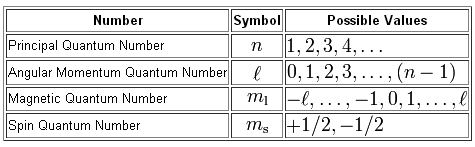Quantum numbers: These four quantum numbers are used to describe the probable location of an electron in an atom.

### The Principal Quantum Number

The first quantum number describes the electron shell, or energy level, of an atom. The value of n ranges from 1 to the shell containing the outermost electron of that atom. For example, in caesium (Cs), the outermost valence electron is in the shell with energy level 6, so an electron in caesium can have an n value from 1 to 6. For particles in a time-independent potential, per the Schrödinger equation, it also labels the nth eigenvalue of Hamiltonian (H) (i.e. the energy E with the contribution due to angular momentum, the term involving J2, left out). This number therefore has a dependence only on the distance between the electron and the nucleus (i.e. the radial coordinate r). The average distance increases with n, thus quantum states with different principal quantum numbers are said to belong to different shells.

### The Azimuthal Quantum Number

The second quantum number, known as the angular or orbital quantum number, describes the subshell and gives the magnitude of the orbital angular momentum through the relation. In chemistry and spectroscopy, ℓ = 0 is called an s orbital, ℓ = 1 a p orbital, ℓ = 2 a d orbital, and ℓ = 3 an f orbital. The value of ℓ ranges from 0 to n − 1 because the first p orbital (ℓ = 1) appears in the second electron shell (n = 2), the first d orbital (ℓ = 2) appears in the third shell (n = 3), and so on. In chemistry, this quantum number is very important since it specifies the shape of an atomic orbital and strongly influences chemical bonds and bond angles.

### The Magnetic Quantum Number

The magnetic quantum number describes the energy levels available within a subshell and yields the projection of the orbital angular momentum along a specified axis. The values of mℓ range from − to ℓ, with integer steps between them. The s subshell (ℓ = 0) contains one orbital, and therefore the mℓ of an electron in an s subshell will always be 0. The p subshell (ℓ = 1) contains three orbitals (in some systems depicted as three “dumbbell-shaped” clouds), so the mℓ of an electron in a p subshell will be −1, 0, or 1. The d subshell (ℓ = 2) contains five orbitals, with m values of −2, −1, 0, 1, and 2. The value of the m quantum number is associated with the orbital orientation.

### The Spin Projection Quantum Number

The fourth quantum number describes the spin (intrinsic angular momentum) of the electron within that orbital and gives the projection of the spin angular momentum (s) along the specified axis. Analogously, the values of ms range from −s to s, where s is the spin quantum number, an intrinsic property of particles. An electron has spin s = ½, consequently ms will be ±, corresponding with spin and opposite spin. Each electron in any individual orbital must have different spins because of the Pauli exclusion principle, therefore an orbital never contains more than two electrons.

For example, the quantum numbers of electrons from a magnesium atom are listed below. Remember that each list of numbers corresponds to (n, l, ml, ms).

Two s electrons: (1, 0, 0, +½) (1, 0, 0, -½)

Two s electrons: (2, 0, 0, +½) (2, 0, 0, -½)

Six p electrons: (2, 1, -1, +½) (2, 1, -1, -½) (2, 1, 0, +½) (2, 1, 0, -½) (2, 1, 1, +½) (2, 1, 1, -½)

Two s electrons: (3, 0, 0, +½) (3, 0, 0, -½)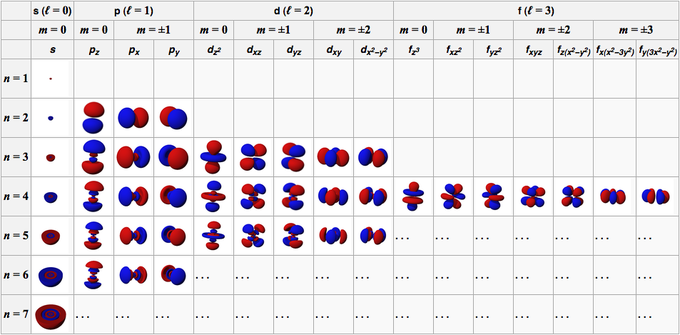Table relating quantum numbers to orbital shape: The relationship between three of the four quantum numbers to the orbital shape of simple electronic configuration atoms up through radium (Ra, atomic number 88). The fourth quantum number, the spin, is a property of individual electrons within a particular orbital. Each orbital may hold up to two electrons with opposite spin directions.

## The Pauli Exclusion Principle

The Pauli exclusion principle states that no two fermions can have identical wavefunctions.

### Learning Objectives

Illustrate how the Pauli exclusion principle partially explains the electron shell structure of atoms.

### Key Takeaways

#### Key Points

• No two identical fermions (particles with half- integer spin) may occupy the same quantum state simultaneously.
• No two electrons in a single atom can have the same four quantum numbers.
• Particles with integer spin occupy symmetric quantum states, and particles with half-integer spin occupy antisymmetric states.

#### Key Terms

• electron: The subatomic particle having a negative charge and orbiting the nucleus; the flow of electrons in a conductor constitutes electricity.
• fermion: A particle with totally antisymmetric quantum states. They have half-integer spin and include many elementary particles.
• boson: A particle with totally symmetric quantum states. They have integer spin and include many elementary particles, and some (gauge bosons) are known to carry the fundamental forces.

The Pauli exclusion principle, formulated by Austrian physicist Wolfgang Pauli in 1925, states that no two fermions of the same kind may simultaneously occupy the same quantum state. More technically, it states that the total wave function for two identical fermions is antisymmetric with respect to exchange of the particles. For example, no two electrons in a single atom can have the same four quantum numbers; if n, ℓ, and mare the same, ms must be different such that the electrons have opposite spins.

The Pauli exclusion principle governs the behavior of all fermions (particles with half-integer spin), while bosons (particles with integer spin) are not subject to it. Fermions include elementary particles such as quarks (the constituent particles of protons and neutrons ), electrons and neutrinos. In addition, protons and neutrons (subatomic particles composed from three quarks) and some atoms are fermions and are therefore also subject to the Pauli exclusion principle. Atoms can have different overall spin, which determines whether they are fermions or bosons—for example, helium-3 has spin 1/2 and is therefore a fermion, in contrast to helium-4 which has spin 0, making it a boson. As such, the Pauli exclusion principle underpins many properties of everyday matter from large-scale stability to the chemical behavior of atoms including their visibility in NMR spectroscopy.

Half-integer spin means the intrinsic angular momentum value of fermions is $\hbar =\frac { h }{ 2\pi }$ (reduced Planck’s constant) times a half-integer (1/2, 3/2, 5/2, etc.). In the theory of quantum mechanics, fermions are described by antisymmetric states. In contrast, particles with integer spin (bosons) have symmetric wave functions; unlike fermions, bosons may share the same quantum states. Bosons include the photon, the Cooper pairs (responsible for superconductivity), and the W and Z bosons. Fermions take their name from the Fermi–Dirac statistical distribution that they obey, and bosons take their name from Bose–Einstein distribution.

### The Exclusion Principle and Physical Phenomena

The Pauli exclusion principle explains a wide variety of physical phenomena. One particularly important consequence of the principle is the elaborate electron-shell structure of atoms and the way atoms share electrons. It explains the variety of chemical elements and their chemical combinations. An electrically neutral atom contains bound electrons equal in number to the protons in the nucleus. Electrons, being fermions, cannot occupy the same quantum state, so electrons have to “stack” within an atom—they have different spins while at the same place.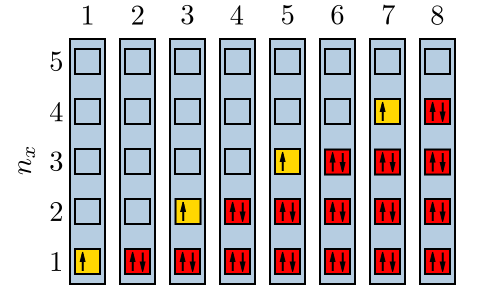Electrons filling quantum energy levels: When a state has only one electron, it could be either spin-up or spin-down. However, according the the Pauli Exclusion Principle, when there are two in a state, there must be one of each.

An example is the neutral helium atom, which has two bound electrons, both of which can occupy the lowest- energy (1s) states by acquiring opposite spin. As spin is part of the quantum state of the electron, the two electrons are in different quantum states and do not violate the Pauli exclusion principle. However, there are only two distinct spin values for a given energy state. This property thus mandates that a lithium atom, which has three bound electrons, cannot have its third electron reside in the 1s state; it must occupy one of the higher-energy 2s states instead. Similarly, successively larger elements must have shells of successively higher energy. Because the chemical properties of an element largely depend on the number of electrons in the outermost shell, atoms with different numbers of shells but the same number of electrons in the outermost shell still behave similarly. For this reason, elements are defined by their groups and not their periods.

## Particle in a Box

The particle in a box model provides one of the very few problems in quantum mechanics which can be solved analytically.

### Learning Objectives

Describe the features of the wave function for the particle in a box.

### Key Takeaways

#### Key Points

• The model is mainly used as a hypothetical example to illustrate the differences between classical and quantum systems.
• The particle may only occupy certain positive-integer energy levels.
• The particle is more likely to be found at certain positions than at others.
• The particle may never be detected at certain positions, known as spatial nodes.

#### Key Terms

• wavefunction: A mathematical function that describes the propagation of the quantum mechanical wave associated with a particle (or system of particles), related to the probability of finding the particle in a particular region of space.
• probability: A number, between 0 and 1, expressing the precise likelihood of an event happening. In this context, the probability of finding a particle at a given position is of interest and is related to the square of the wave function.

Also called the infinite square well problem, the particle in a box is one of the very few problems in quantum mechanics that can be solved without approximations.

The solution to the particle in a box can be found by solving the Schrödinger equation:

$-\frac { { \hbar }^{ 2 } }{ 2m } \frac { { \partial }^{ 2 }\Psi }{ { \partial x }^{ 2 } } +V(x)\Psi \quad =\quad i\hbar \frac { \partial \Psi }{ \partial t }$

The potential function (V) is time-independent, while the wavefunction itself is time- dependent.

The infinite square well is defined by a potential function in which the potential $V(x)$ is 0 for values of x between 0 and L, where L is the length of the box, and infinite at all other locations. In this case, classical and quantum physics are describing the same thing: the impossibility of a particle “leaping” over an infinitely high boundary at the edge of the container.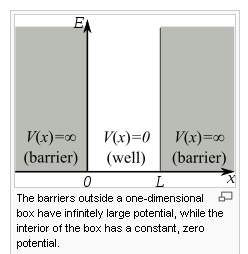The potential well: Energy and position relationships of the particle in a box. Inside the box the potential V(x) is zero. Outside the box the potential energy is infinite.

Separating the variables reduces the problem to one of simply solving the spatial part of the equation:

$-\frac { { \hbar }^{ 2 } }{ 2m } \frac { { d }^{ 2 }\psi }{ { dx }^{ 2 } } +V(x)\psi{(x)} \quad =\quad E\psi{(x)}$

E represents the possible energies that can describe the system. The above equation establishes a direct relationship between the second derivative of the the wave function and the kinetic energy of the system. The best way to visualize the time-independent Schrödinger equation is as a stationary snapshot of a wave at particular moment in time.

No forces act on the particle inside of a box, which means that the part of the wave function between 0 and L can oscillate through space and time with the same form as a free particle:

$\psi (x,t)\quad =\quad [A\sin { (kx) } +\quad B\cos { (kx)]{ e }^{ -i\omega t } }$

B and A are arbitrary complex numbers. The frequency of the oscillations through space and time are given by the wave number, $k$, and the angular frequency, $\omega$ respectively. The probability of finding the particle at the edge of the box is 0 because the potential there is infinite; the cosine term, therefore, can be removed to leave only the sine term. Differential calculus then reveals that the energy of the particle is given by:

$E=\hbar \omega =\frac { { \hbar }^{ 2 }{ k }^{ 2 } }{ 2m }$

The size or amplitude of the wave function at any point determines the probability of finding the particle at that location, as given by the equation:

$P(x,t)={ \left\lfloor \psi (x,t) \right\rfloor }^{ 2 }$

The wavefunction must vanish everywhere beyond the edges of the box, as the potential outside of the box is infinite. Furthermore, the amplitude of the wavefunction also may not “jump” abruptly from one point to the next. These two conditions are only satisfied by wavefunctions with the form:

${ \psi }_{ n }(x,t)\quad =\quad A\sin { { (k }_{ n } } x){ e }^{ -i{ \omega }_{ n }t }$

because wavefunctions based on sine waves will have Ψ(x) = 0 values when x = o and x = L, while those wave functions which include cosine terms will not.

${ k }_{ n }\quad =\quad \frac { n\pi }{ L }$

where n = {1,2,3,4…} and L is the size of the box. Negative values are neglected, since they give wavefunctions identical to the positive solutions except for a physically unimportant sign change. Finally, the unknown constant may be found by normalizing the wavefunction so that the total probability density of finding the particle in the system is 1.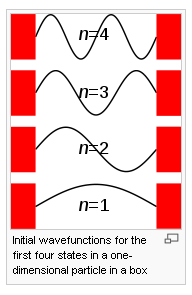Solutions to the particle in a box problem: The first four solutions to the one dimensional particle in a box. Note that just like a guitar string, the solutions to the particle in a box problem are constrained to those wavefunctions that anchor the amplitude at the walls of the box as zero.

Normalizing, we get $\left| A \right| =\sqrt { \frac { 2 }{ L } }$

A may be any complex number with absolute value $\left| A \right| =\sqrt { \frac { 2 }{ L } }$

When treated as a probability density, the square of the wave function (Ψ2) describes the probability of finding the particle at a given point and at a given time. Four conditions, proposed by Max Born, must be met for this to be true:

1. The wave function must be single-valued.
2. The wave function must be “square integrable.”
3. The wave function must be continuous everywhere.
4. The first derivative of the wave function must be continuous.

## Wave Equation for the Hydrogen Atom

The hydrogen atom is the simplest one-electron atom and has analytical solutions to the Schrödinger equation.

### Learning Objectives

Explain how the solution of the Schrödinger equation for the hydrogen atom yields the four quantum numbers and use these to identify degenerate states

### Key Takeaways

#### Key Points

• The Schrödinger equation is a differential equation that provides a way to calculate the possible wavefunctions of a system and describes how they dynamically change in time.
• Particles can behave like waves because their corresponding wavefunction satisfies the Schrödinger equation.
• The motion of a particle, described by a wave packet solution to the Schrödinger equation, is also described by the Hamilton–Jacobi equation of motion.

#### Key Terms

• Hamiltonian: In quantum mechanics, the observable, denoted by H, that corresponds to the total energy of the system.
• quantum number: One of certain integers or half-integers that specify the state of a quantum mechanical system (such as an electron in an atom).
• eigenfunctions: A class of mathematical function that is non-zero and returns the same function except for a multiplicative scaling factor when acted on by a linear operator (such as a Hamiltonian).

The solution of the Schrödinger equation for the hydrogen atom uses the fact that the Coulomb potential produced by the nucleus is isotropic—it is radially symmetric in space and only depends on the distance to the nucleus. Although the resulting energy eigenfunctions (the orbitals ) are not necessarily isotropic themselves, their dependence on the angular coordinates follows generally from this isotropy of the underlying potential. The eigenstates of the Hamiltonian (that is, the energy eigenstates) can be chosen as simultaneous eigenstates of the angular momentum operator. This corresponds to the fact that angular momentum is conserved in the orbital motion of the electron around the nucleus. Therefore, the energy eigenstates may be classified by two angular momentum quantum numbers, ℓ and m(both are integers). The angular momentum quantum number ℓ = 0, 1, 2,… determines the magnitude of the angular momentum. The magnetic quantum number m= −,…, +ℓ determines the projection of the angular momentum on the (arbitrarily chosen) z-axis and therefore the orientation of the orbital in three-dimensional space.

In addition to mathematical expressions for total angular momentum and angular momentum projection of wavefunctions, an expression for the radial dependence of the wavefunctions must be found. It is only here that the details of the 1/r Coulomb potential enter (leading to Laguerre polynomials in r). This leads to a third quantum number, the principal quantum number n = 1, 2, 3,…. The principal quantum number in hydrogen is related to the atom’s total energy. Note that the maximum value of the angular momentum quantum number is limited by the principal quantum number: it can run only up to n − 1, i.e. ℓ = 0, 1,…, n − 1.

### Degeneracy of Different Magnetic Quantum Numbers

Due to angular momentum conservation, states of the same ℓ but different mhave the same energy. This holds for all problems with rotational symmetry. For the hydrogen atom, states of the same n but different ℓ are also degenerate (they have the same energy). This is a specific property of hydrogen and is not true for more complicated atoms. These atoms have an effective potential differing from the 1/r form due to the presence of the inner electrons shielding the nucleus potential.

The spin of the electron adds the last quantum number, the projection of the electron’s spin angular momentum along the z-axis, which can take on two values. Therefore, any eigenstate of the electron in the hydrogen atom is described fully by four quantum numbers. According to the usual rules of quantum mechanics, the actual state of the electron may be any superposition of these states. This explains also why the choice of z-axis for the directional quantization of the angular momentum vector is immaterial: an orbital of given ℓ and m′ obtained for another preferred axis, z′,can always be represented as a suitable superposition of the various states of different m (but same ℓ) that have been obtained for z.

Using a three-dimensional approach, the following form of the Schrödinger equation can be used to describe the hydrogen atom:

$E\psi=-\frac{\hbar^{2}}{2\mu}\nabla^2\psi-\frac{e^2}{4\pi\epsilon_0r}\psi$

Schroedinger Equation: Three dimensional Schrödinger equation as applied to the H atom.

where $\mu$ is the reduced mass of the electron-proton pair. The wavefunction itself is expressed in spherical polar coordinates:

$\psi \left( r,\theta,\phi \right) =R\left( r \right) { Y }_{ l }^{ m }\left( \theta,\phi \right) =R\left( r \right) \Theta \left( \theta \right) \Phi \left( \phi \right)$

where R are radial functions and theta ($\theta$) and phi ($\phi$) are spherical harmonic terms. The generalized family of solutions is:

${\psi }_{ n,l,m }\left( r,\theta,\phi \right) =\sqrt { { \left( \frac { 2 }{ n{ a }_{ 0 } } \right) }^{ 3 }\frac { \left( n-l-1 \right) ! }{ { 2n[(n+l)! ] }^{ 3 } } } { e }^{ { -r }/{ { na }_{ 0 } } }{ (\frac { 2r }{ n{ a }_{ 0 } } ) }^{ l }{ L }_{ n-l-1 }^{ 2l+1 }(\frac { 2r }{ n{ a }_{ 0 } } )\cdot { Y }_{ l }^{ m }(\theta,\phi )$

where a0 is the Bohr radius, L are the generalized Laguerre polynomials, and n, l, and m are the principal, azimuthal, and magnetic quantum numbers, respectively.